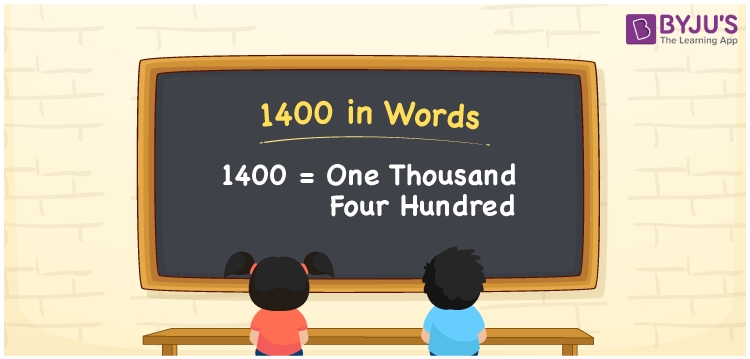# 1400 in Words

We can write 1400 in words as One thousand four hundred. Suppose you bought a new gadget that costs Rs. 1400, then you could say that “ I bought a new gadget for one thousand four hundred rupees”. In this article, you will learn how to convert the number 1400 into words in an exciting way.

 1400 in words One thousand four hundred One thousand four hundred in Numbers 1400

## 1400 in English Words

We generally write numbers in words using the English alphabet. Thus, we can read 1400 in English as “One thousand four hundred”.## How to Write 1400 in Words?

In this section, you will learn how to write the number 1400 in words. The given number 1400 has four digits, so we should make a place value chart that represents the place value for all these four digits. The order of place values of digits in a number is in accordance with the Indian numbering system, such as:

• Ones
• Tens
• Hundreds
• Thousands
• Ten-thousands
• Hundred-thousands or Lakhs

This can be done as follows.

 Thousands Hundreds Tens Ones 1 4 0 0

Thus, we can write the expanded form as:

1 × Thousand + 4 × Hundred + 0 × Ten + 0 × One

= 1 × 1000 + 4 × 100 + 0 × 10 + 0 × 1

= 1400

= One thousand five hundred

1400 is a natural number that precedes 1401 and succeeds 1399.

1400 in words – One thousand five hundred

Is 1400 an odd number? – No

Is 1400 an even number? – Yes

Is 1400 a perfect square number? – No

Is 1400 a perfect cube number? – No

Is 1400 a prime number? – No

Is 1400 a composite number? – Yes

## Frequently Asked Questions on 1400 in Words

Q1

### How do you write 1400 in English?

We can write 1400 in English words as “One thousand four hundred”.
Q2

### How do you write 1400 in words for a cheque?

For a cheque, we generally write 1400 in words as One thousand four hundred rupees only.
Q3

### What number is 1400 in words?

The number 1400 is One thousand four hundred in words.